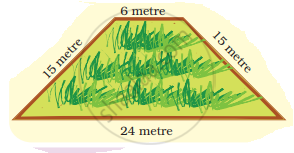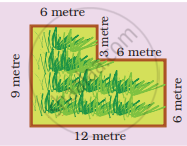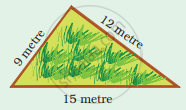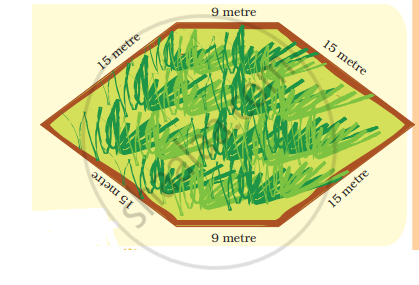# Here are pictures of some more fields. Find out which one has the longest boundary? Boundary = ______ metre Boundary = ______ metre Boundary = ______ metre Boundary = ______ metre - Mathematics

Numerical

Here are pictures of some more fields. Find out which one has the longest boundary?

1.Boundary = ______ metre

2.Boundary = ______ metre

3.Boundary = ______ metre

4.Boundary = ______ metre

#### Solution

1. Length of boundary = 15 metre + 6 metre + 15 metre + 24 metre
= 60 metre
2. Boundary = 9 metre + 6 metre + 3 metre + 6 metre + 6 metre + 12 metre
= 42 metre
3. Boundary = 9 metre + 6 metre + 3 metre + 6 metre + 6 metre
+ 12 metre
= 42 metre
4. Boundary = 15 metre + 15 metre + 9 metre + 15 metre + 15 metre + 9 metre
= 78 metre

Therefore, field (d) has the longest boundary.

Concept: Fields and Fences
Is there an error in this question or solution?

#### APPEARS IN

NCERT Mathematics - Math - Magic Class 4 CBSE
Chapter 13 Fields and Fences
Q 1. | Page 151